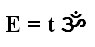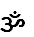﻿ TIKOV.COM » Sciencenow its build 1.1 24.06 2012

Theory of Physics of Time .

"Possibility of Time Reactor"

First of all i want to introduce you some formulaExplanation: E is energy t is time andis ancient symbol OM and in this formula it means vibration (vibe), some process that starts and ends in time, some activity that envolves atoms and moleculas to act for example as alive creature, physical process, sound, image or work with information. I decided not to follow scientific tradition of using greek alphabet for formulas and decided to use ancient symbol from Ancient India tradition. This combination as i think, can create some good base for proper understanding of concepts that are shown by this formula.

Lets try to understand what this formula means. The best way to understand it is to create a bridge between past and future, and to connect this formula to previous formulas.

Very famous formula of Einstein tells us that E= mc² or energy is mass of object multiplied by speed of light multiplied on itself. (Probably some of you knows that light is one type of vibration (vibe))

Lets connect my formula and Einstein

We have vibration elementat one side and vibration element c² on the other side= mc² (Sorry for harsch design, ok?)

So now we can define whatmeans= mc² \ t = E\t

So "The Vibe"is energy in a measure of time and its measured as Omm ( Its not Om from Greek alphabet used in Elecricity it has two m`s - Omm)

Would they give me Nobel Prize after that? Because this formula opens tremendous possibilities?

Jeje. i guess not, there is not so many pages, so i continue soon.

Coming Soon:

Positive and negative vibes - if we understand this two types of vibes what it will bring to humanity?

What is Time?

Social Physics Research Project is on the way